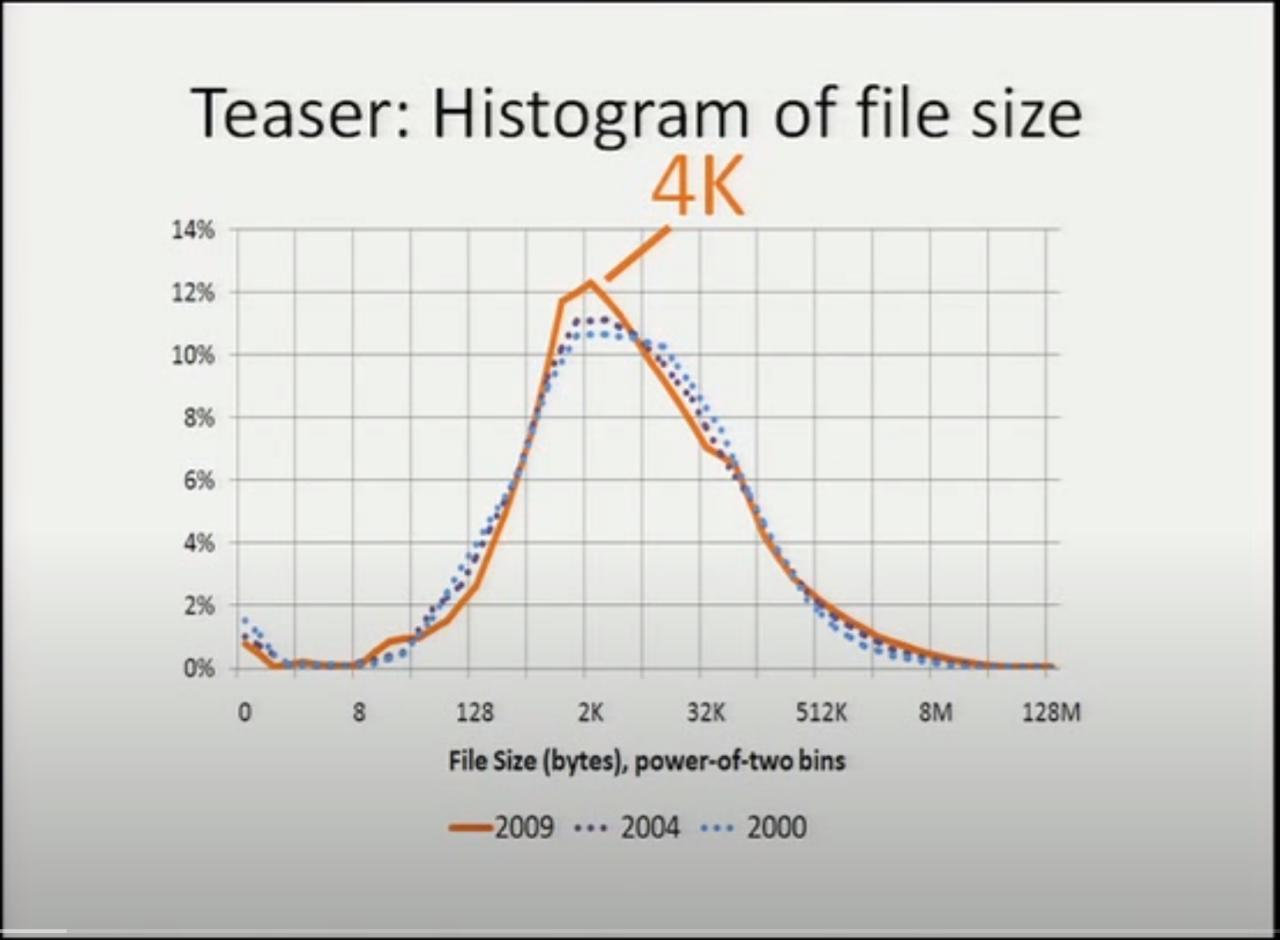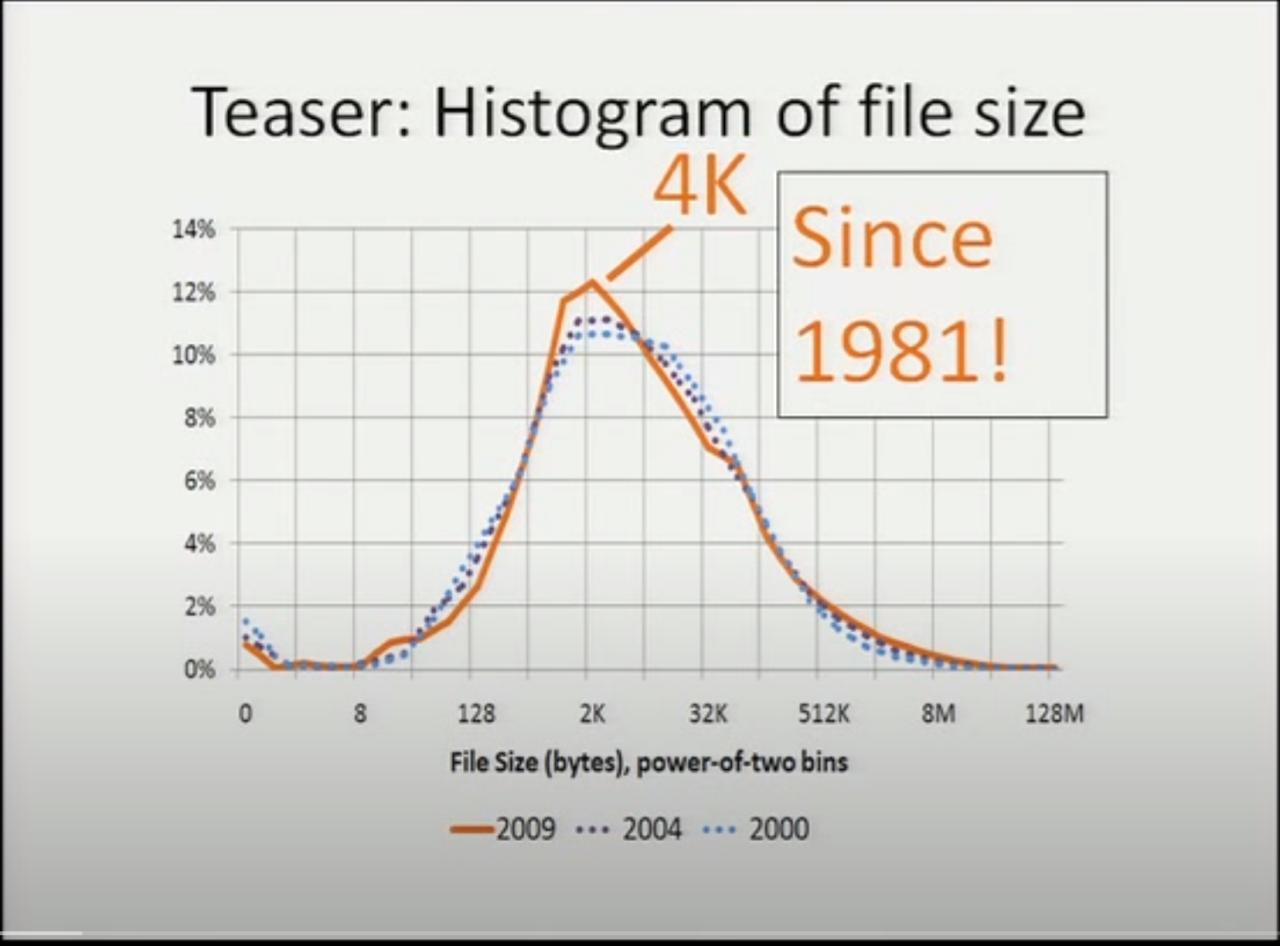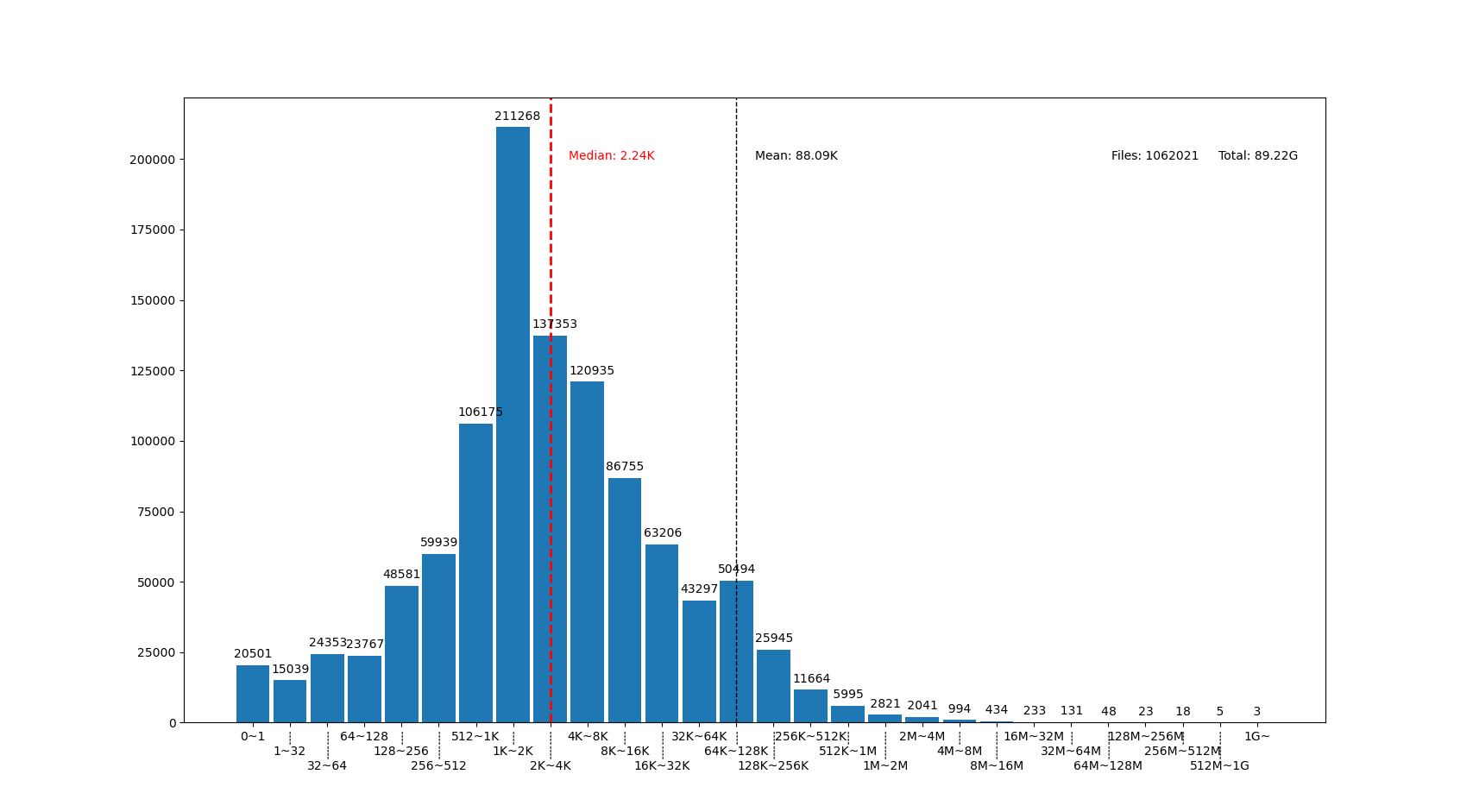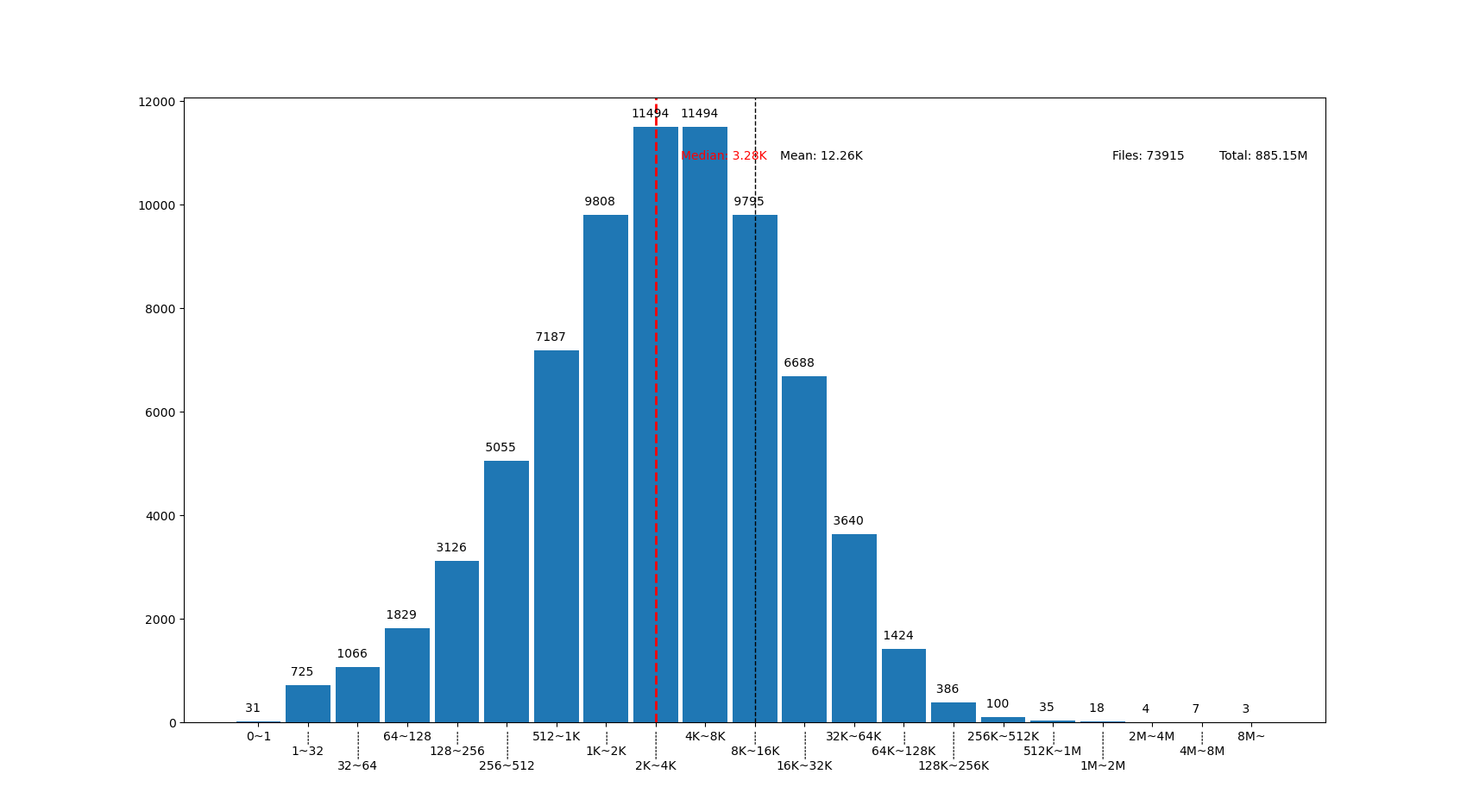# 系统中的大多数文件有多大？

## 你觉得，你的系统中大多数文件大概有多大？

• 我收藏了好多照片，每个有 2~5MiB 吧。
• 我下载了好多漫画，每个 100KiB 左右，这些大概占了不少比例。
• 我还收藏了不少动画电影电视剧，虽然这些文件总数量可能不多？
• 我下载了 Linux 的源码，那里面每个 C 代码文件都几千行，每行 100 字宽，平均也得有 30KiB 吧，有几万个源码文件呢，占比应该挺大的……

• 统计学上说，大量独立随机事件的累积概率满足正态分布（常态分布）曲线。假设我们把某个特定文件的大小增长 1字节看作是一次独立随机事件，那么文件大小在文件系统中应该是满足正态分布的？
• 正态分布的前提下，平均数接近中位数，文件系统的已占用大小除以文件数量大概就是大部分文件的大小了吧。
• 根据我现在文件系统的占用大小和文件数量，平均数大概是 500KiB 左右？
• 虽然我还存了几个非常大，上 GiB 的文件，但是看起来似乎也有很多很多非常小的文件， 平均一下的话应该会把平均数拉大，大于中位数吧。那么中位数应该在 100KiB 这样的量级附近？

「我有个仓库盘要存很多下载到的漫画，每个漫画都是一个文件夹里面一堆 小 JPG ，每个就几十 KiB 。网上看到的说法是 XFS 对 小文件 的性能不那么好，我是不是该换 EXT4 ？我还想在 Windows 上能读写，是不是 ExFAT 这种简单的文件系统更合适一点？」

「软件源的镜像服务器需要存的都是些 小文件 吧，大多数软件包压缩后也就是几个 KiB 到几个 MiB 的量级，这种需求是不是适合用对 小文件 优化比较好的文件系统？」

「我的程序需要分析的数据是大量几百K的 小文件 ，该怎么存合适呢，直接用文件系统还是应该上数据库？ 我还想多线程并发分析，是不是 SQL 数据库的并发能力强一些？又或者 MongoDB 的 GridFS 看起来似乎能结合文件系统和数据库的特点，选它应该还不错？」

## 统计实际系统中文件大小的学术研究…… the median file size is 4k. It was 4k the other two years of the study. We've actually gone back through the literature. It turns out it's 4k in every study going back to the last 30 years. So this is great news. We can finally compete with physicists: we have our own fundamental constant of the universe, it's a medium file size ……

## 不信？你可以测一下自己的文件系统

```find /home -type f -printf "%s %p\n" > myhome.txt
```

```#!/usr/bin/python3
import argparse
import matplotlib.pyplot as plt
import numpy as np
import sys
from math import *
from bisect import bisect_left

def numfmt(s):
marks = "KMGTP"
m = 0
f = type(s) is float
while s >= 1024 and m < len(marks):
if f:
s /= 1024.0
else:
s //=1024
m += 1
if f:
return f"{s:.2f}{marks[m-1:m]}"
else:
return f"{s}{marks[m-1:m]}"

if __name__ == '__main__':
parser = argparse.ArgumentParser(
prog = "filesizehistogram",
description = """
can use "-" as input filename, indicate input is taken from stdin.
otherwise input file should be a result of "find -printf \'%s %p\\n\'"
"""
)
parser.add_argument('-o', '--output', help="output filename, will recognize common extensions by matplot")
args = parser.parse_args()

filenames = [x if x != '-' else '/dev/stdin' for x in args.input]
data=np.array([int(x.split(' ')) for fn in filenames for x in open(fn)])
mindatalog2 = 5 # cut from 32
maxdatalog2 = min(ceil(log2(data.max())), 31) # cut at 1G and above
# bins [0, 1, 32, 64, 128, 256, ... , 1G, 2G] , last bin is open range
bins=[0,1,] + [2**x for x in range(mindatalog2, maxdatalog2 + 1)]

median = float(np.median(data))
mean = float(data.mean())
bmedian = bisect_left(bins, median) - 1
bmean = bisect_left(bins, mean) - 1
files = len(data)
total = data.sum()

hist, bin_edges = np.histogram(data,bins)
fig,ax = plt.subplots(figsize=(20,8))
ax.bar(range(len(hist)), hist, width=0.9)
ax.set_xticks([i for i in range(len(hist))])
tickbar = "┊\n"
ax.set_xticklabels([f'{tickbar*(i%3)}{numfmt(bins[i])}~{numfmt(bins[i+1])}' for i in range(len(hist)-1)] +
[f"{numfmt(bins[len(hist)-1])}~"])

ax.axvline(bmean, color='k', linestyle='dashed', linewidth=1)
ax.axvline(bmedian, color='r', linestyle='dashed', linewidth=2)
min_ylim, max_ylim = plt.ylim()
min_xlim, max_xlim = plt.xlim()
ax.text(bmean + 0.5   , max_ylim * 0.9, f'Mean: {numfmt(mean)}')
ax.text(bmedian + 0.5 , max_ylim * 0.9, f'Median: {numfmt(median)}', color='r')
ax.text(max_xlim * 0.8, max_ylim * 0.9, f'Files: {files}')
ax.text(max_xlim * 0.9, max_ylim * 0.9, f'Total: {numfmt(float(total))}')

for i in range(len(hist)):
ax.text(i - 0.5, hist[i] + files / 400, f"{hist[i]:5}") # label on top of every bar, uplefted a little

if args.output:
plt.savefig(args.output)
else:
plt.show()
```linux 代码树的文件大部分比我猜的 30K 要小呢，主要在 1K~16K ，中位数 3.28K 。而且意外得在代码树里有好几个 0 大小的文件，看了几个文件路径确认了一下，它们的确是 0 大小的头文件，并不是我的文件系统丢了文件内容。